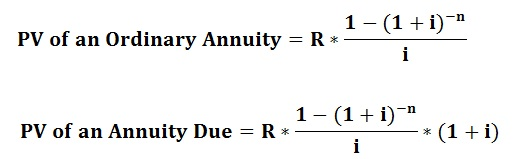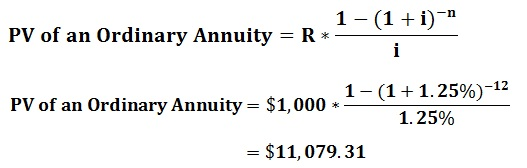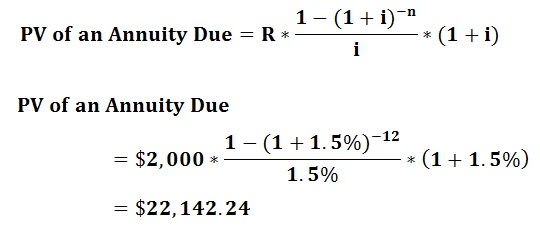# How to Calculate Present Value of an Annuity

## Ordinary Annuity and Annuity Due

An annuity can be considered as a way of making equal payments for a specific period and it can be divided into two types as ordinary annuity and annuity due. According to the ordinary annuity, periodic payments are being made at the end of the period. A good example of an ordinary annuity is the interest payments from bond issues. Another example which can be used is the present value of cash flows from an investment. Both these payments are usually made at the end of a period.

In the annuity due, periodic payments need to be made at the beginning of the period. A very good example is the lease payments made by the business organizations. If a company purchases any fixed asset (equipments, buildings) lease payments need to be made at the first day of the month. i.e. annuity due.

## What is Present Value of An Annuity?

The addition of the periodic payments which are being discounted at a specific interest rate can be referred as the present value of an annuity. It is based on the concept of the time value of money, which means that the value of money depreciates with time due to inflation, fluctuations in exchange rates, etc. Therefore, the worth of cash at present is more than in future periods.

## Formulas to calculate the Present Value of an annuity

Following formulas can be used in order to calculate present value of an annuity, which is the present value of the ordinary annuity and the present value of the annuity due.Where,

i = Interest rate per compounding period
n = The number of compounding periods
R = Fixed periodic payment

For example, when calculating the present value on 01/01/2013 of the annuity of \$ 1,000 paid at the end of each month of the year 2013, with the interest rate of 15%, calculation can be presented as follows:

R = \$1000
n = 12
i = 15%/12 = 1.25%Another example is that a certain amount was invested on 01/01/2013, which generates \$2,000 at the beginning of every month in year 2013. The interest rate on the investment was 18%. The original investment and the interest earned can be calculated as follows:

R = \$2,00
n = 12
i = 18%/12 = 1.5%

Original Investment = PV of annuity due on 01/01/2013Earned amount of interest = [(\$2,000*12) – \$22,142.24] =\$1,857.76

According to the type of the situation, the most related formula needs to be applied to calculate present value of an annuity.